# Resultant Velocity Power Spectral Density Calculation

edited June 30

Full Video of Analysis: https://info.endaq.com/hubfs/psd_velocity.mp4

Related article on data ("guess the vibe").

## Time Data

First here is the time series data, the resultant is calculated with a root sum of squares = (x^2+y^2+z^2)^0.5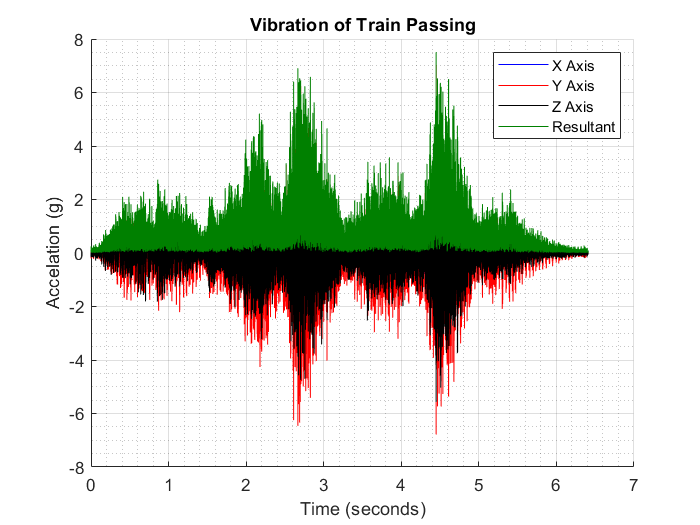Here it is zoomed in, still very messy and hard to understand!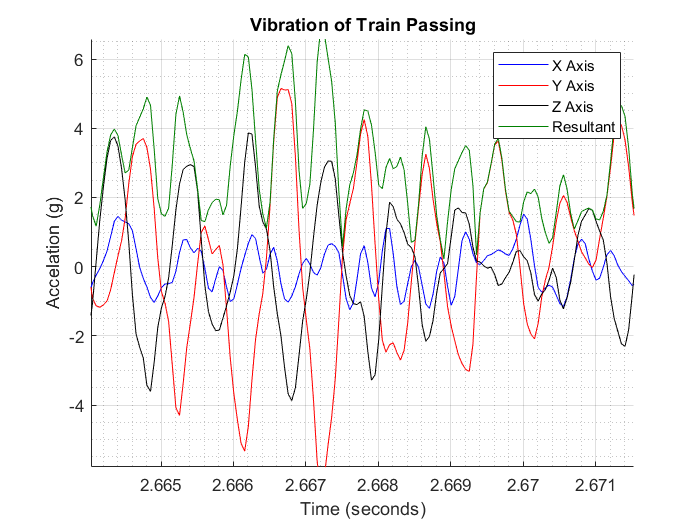## Power Spectral Density

These initial PSDs are "messy" because of the small bin width.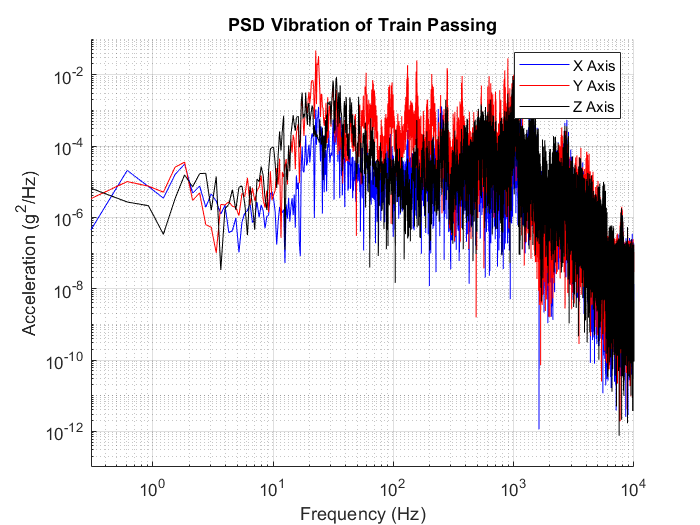But they can be converted to 1/3 octave spacing (each frequency point is x2^(1/3) compared to the previous). In this cleaner format, the root sum of the squares can also be determined for a resultant PSD.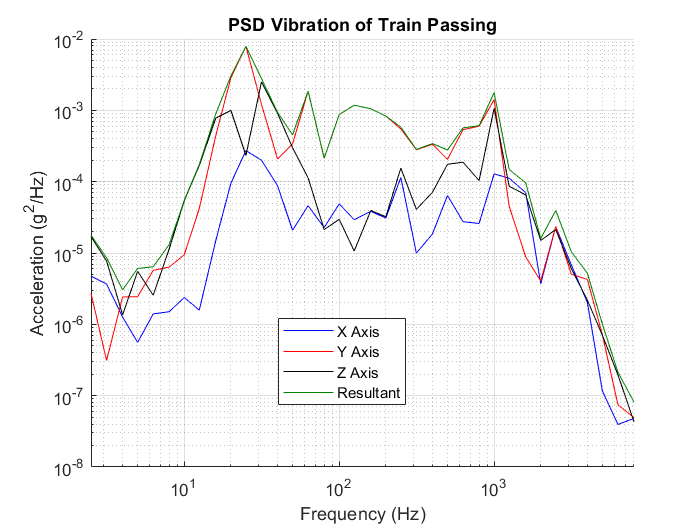And now this can be converted to velocity easily in the frequency domain, attached is a PDF from VibrationResearch which goes into more detail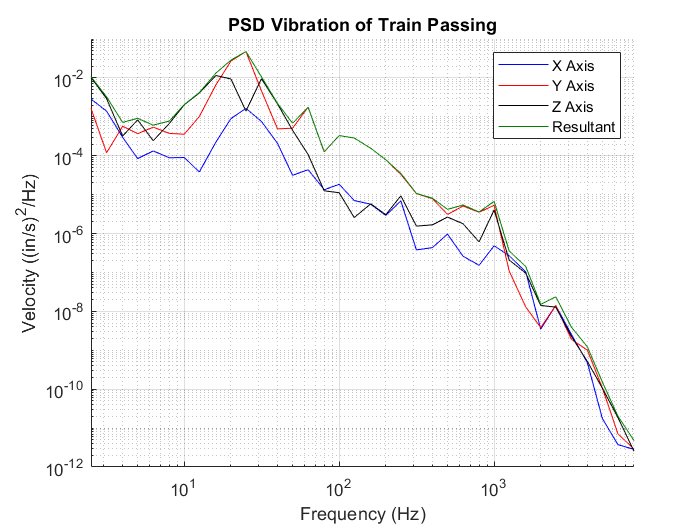Tagged: